Courses

# Test: Critical Phenomenon

## 16 Questions MCQ Test Chemistry for JEE | Test: Critical Phenomenon

Description
This mock test of Test: Critical Phenomenon for JEE helps you for every JEE entrance exam. This contains 16 Multiple Choice Questions for JEE Test: Critical Phenomenon (mcq) to study with solutions a complete question bank. The solved questions answers in this Test: Critical Phenomenon quiz give you a good mix of easy questions and tough questions. JEE students definitely take this Test: Critical Phenomenon exercise for a better result in the exam. You can find other Test: Critical Phenomenon extra questions, long questions & short questions for JEE on EduRev as well by searching above.
QUESTION: 1

### Direction (Q. Nos. 1-3) This section contains 3 multiple choice questions. Each question has four choices (a), (b), (c) and (d), out of which ONLY ONE option is correct. Q. The critical tem perature of water is higher than that of O2 because the H2O molecules have

Solution:

Critical temperature is directly proportional to intermolecular force of attraction. H2O is a polar molecule, has greater intermolecular force of attraction than O2, hence higher critical temperature.

QUESTION: 2

Solution:
QUESTION: 3

###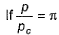(reduced pressure),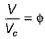(reduced volume) and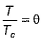(reduced temperature), then,

Solution:

Let P/PC = π, V/VC = Φ and T/TC = θ
(P + a/V2)(V-b) = RT
(πPC + a/Φ2VC2)(ΦVC - b) = RT
(πa/27b2 + a/Φ29b2)(3Φb - b) = R×8θa/27Rb
a/27b(π+3/Φ2)(3Φ-1) = 8θa/27b
(π + 3/Φ2)(3Φ-1) = 8θ

QUESTION: 4

Direction (Q. Nos. 4) Choice the correct combination of elements and column I and coloumn II  are given as option (a), (b), (c) and (d), out of which ONE option is correct.

Q. Values of different types of temperatures have been given in Column II. Match these values with the correct definition of temperatures in Column I and mark the correct answer from codes :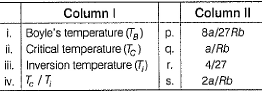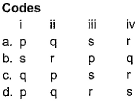Solution:

TC = 8a/27Rb
TB = a/Rb
TI = 2a/Rb
TC/TI = 4/27

*Multiple options can be correct
QUESTION: 5

Direction (Q. Nos. 5-6) This section contains 2 multiple choice questions. Each question has four choices (a), (b), (c) and (d), out of which ONE or  MORE THANT ONE  is correct.

Q. Based on the following isotherms, select correct statements.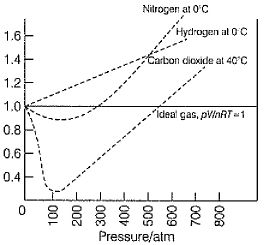Solution:
*Multiple options can be correct
QUESTION: 6

At Boyle’s temperature

Solution:

It is the temperature at which non-ideal gas exhibits almost ideal behaviour for a considerable range of pressure. So to behave as ideal gas, attractive and repulsive forces cancel each other. So option a and b are correct.

QUESTION: 7

Direction (Q. Nos. 7-10) This sectionis based on statement I and Statement II. Select the correct answer from the code given below.

Q.

Statement I : Gases do not liquefy above th e ir Tc (critical temperature), even on applying high pressure.

Statement Il : Above Tc , m olecular speed is high and interm olecular attractions cannot hold the molecules together, because they escape due to high speed.

Solution:

The correct answer is option A
Both statement 1 and statement 2 are true and statement 2 is the correct explanation of statement 1. Gases do not liquefy above their critical temperature, even applying high pressure because above critical temperature, the molecular speed is high and intermolecular attractions cannot hold the molecules together.

QUESTION: 8

Statement I : At Tc , liquid passes into gaseous state im perceptibly and continuously.

Statement II : At Tc, density of liquid and gaseous phase is equal.

Solution:

Both assertion and reason are true and reason is the correct explanation of assertion. Gases do not liquefy above their critical temperature, even an applying high pressure because above critical temperature, the molecular speed is high and intermolecular attractions cannot hold the molecules together.

QUESTION: 9

Statement I : At Tc, there is no distinction between the gaseous state and liquid state and no second phase is formed irrespective of the pressure of the system.

Statement II : At the critical point, densities of a substance in gaseous and liquid states are equal.

Solution:

At critical temperature, there is no distinction b/w liquid and gases irrespective of pressure applied. Critical temperature depends on the magnitude of intermolecular forces of attraction present between the gas molecules.
Higher critical temperature represents strong intermolecular forces of attraction present between the gas molecules.
Such gas can be easily liquified.

QUESTION: 10

Statement I : Reduced equation of state in terms of reduced temperature θ reduced volume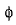and reduced pressure π can be applied to solid, gases and liquid.

Statement II: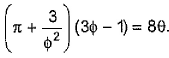Equation is independent of p, V and T as well as independent of van der Waais’ constant a and b.

Solution:

Let P/PC = π, V/VC = Φ and T/TC = θ
(P + a/V2)(V-b) = RT
(πPC + a/Φ2VC2)(ΦVC - b) = RT
(πa/27b2 + a/Φ29b2)(3Φb - b) = R×8θa/27Rb
a/27b(π+3/Φ2)(3Φ-1) = 8θa/27b
(π + 3/Φ2)(3Φ-1) = 8θ

QUESTION: 11

Direction (Q. Nos. 11-16) This section contains 3 paragraph, wach describing theory, experiments, data etc. three Questions related to paragraph have been given.Each question have only one correct answer among the four given options (a),(b),(c),(d).

Passage I

Densities of O2(g) and O2(/) and also their average values are plotted against temperatures.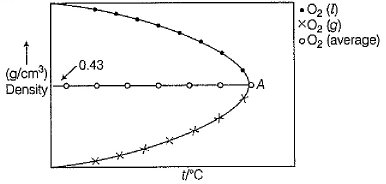Q. The point of intersection is Tc. Based on above graph, critical volume of O2 is

Solution:

In the graph, we can see that at critical temperature, density of (O2) is almost constant.
Therefore, d = constant
MP/RT = constant
M/0.43 = RT/P     (since n = 1)
32/0.43 = VC
VC = 74.4cm3/mol

QUESTION: 12

Passage I

Densities of O2(g) and O2(/) and also their average values are plotted against temperatures.Q. Van der Waais’ constant ‘b' of O2 gas is thus,

Solution:
QUESTION: 13

Passage II

When interm olecular forces in a real gas are taken zero, variation of pV with pressure p at a given temperature Is shown by following isotherm.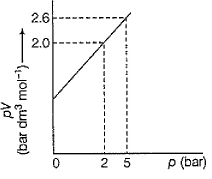Q. Thus, critical volume Vc is

Solution:
QUESTION: 14

Passage II

When interm olecular forces in a real gas are taken zero, variation of pV with pressure p at a given temperature Is shown by following isotherm.Q. If Tc = 100 K fo r this gas then, pc is

Solution:
QUESTION: 15

Passage III

Isotherms of carbon dioxide , I (at T1), II (at T2),

III (at T3) and IV (at T4) are given. Also, T1 < T2 < T3 < T4 .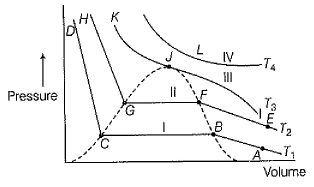Q. Consider the following facts.

I. On increasing volume at T1 or T2 there is sharp decrease in pressure indicating liquefaction.
II. At T3, there is no horizontal curve indicating no liquefaction. Thus, T3 is critical temperature.
III. Along CB or GF, there is liquefaction thus liquid and gaseous phases are present.

Select the correct facts.

Solution:

All statements are correct.
On increasing volume at T1 or T2 there is a sharp decrease in pressure indicating liquefaction. As the temperature is below critical temperature, liquefaction happens there.
At T3, there is no horizontal curve indicating no liquefaction. Thus, T3 is critical temperature. Above T3, the graph doesn’t enter the region on V axis and so no liquefaction is possible.
Along CB or GF, there is liquefaction thus liquid and gaseous phases are present. As the lines are in the region, both liquid and gaseous state co-exists.

QUESTION: 16

Passage III

Isotherms of carbon dioxide , I (at T1), II (at T2),

III (at T3) and IV (at T4) are given. Also, T1 < T2 < T3 < T4 .Q. Complete liquefaction at T1, and T2 takes place respectively at points.

Solution: